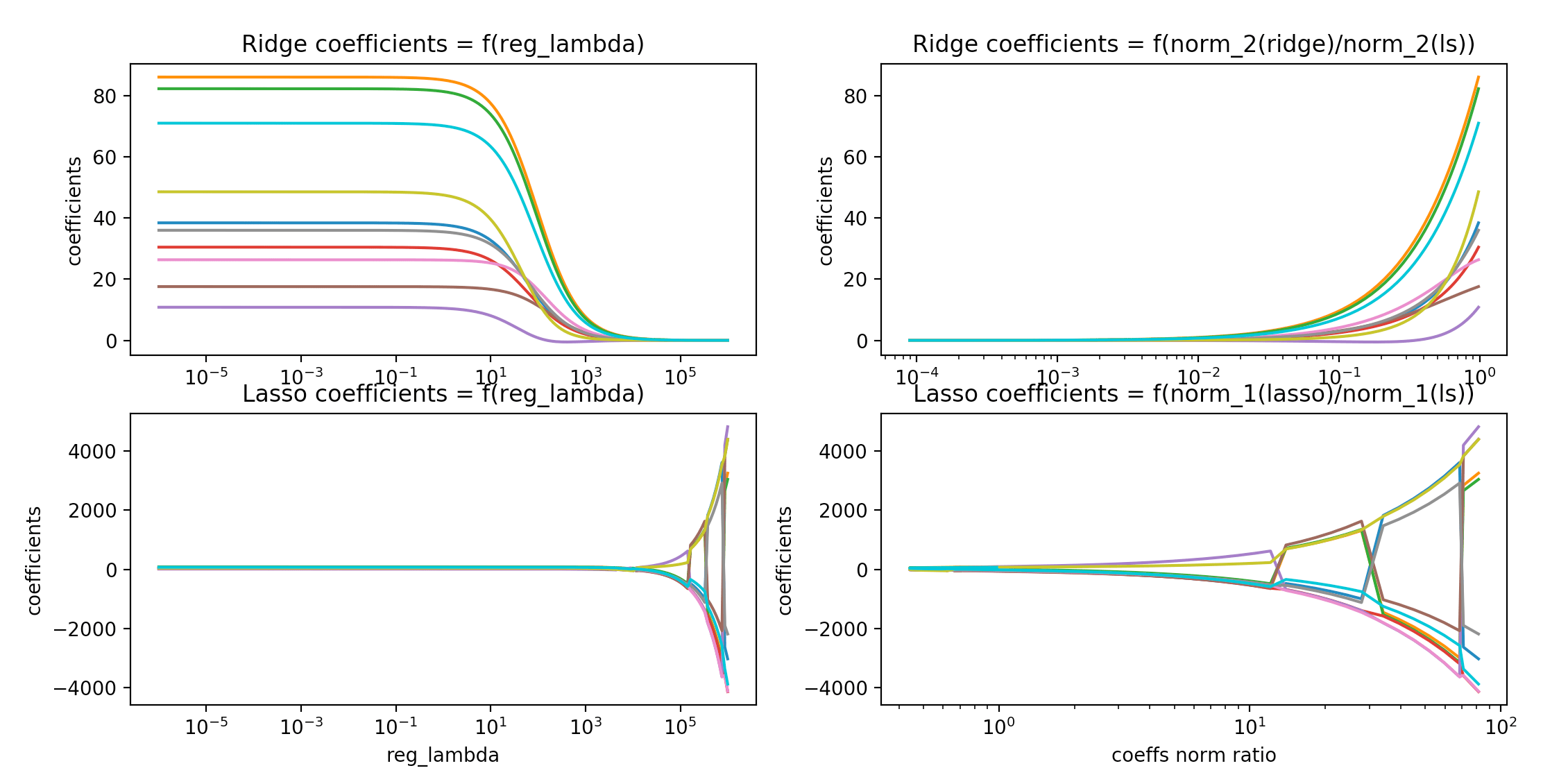This article was first published on T. Moudiki's Webpage - Python , and kindly contributed to python-bloggers. (You can report issue about the content on this page here)

This post is about `LSBoost`, an Explainable ‘AI’ algorithm which uses Gradient Boosted randomized networks for pattern recognition. As we’ve discussed it last week `LSBoost` is a cousin of GFAGBM’s `LS_Boost`. In `LSBoost`, more specifically, the so called weak learners from `LS_Boost` are based on randomized neural networks’ components and variants of Least Squares regression models.

I’ve already presented some promising examples of use of `LSBoost` based on Ridge Regression weak learners. In mlsauce’s version `0.7.1`, the Lasso can also be used as an alternative ingredient to the weak learners. Here is a comparison of the regression coefficients obtained by using mlsauce’s implementation of Ridge regression and the Lasso:# R example: LSBoostRegressor with Ridge regression and the Lasso

The following example is about training set error vs testing set error, as a function of the regularization parameter, both for Ridge regression and Lasso-based weak learners.

## Packages and data

```# 0 - Packages and data -------------------------------------------------------

library(devtools)
devtools::install_github("thierrymoudiki/mlsauce/R-package")
library(mlsauce)
library(datasets)

print(summary(datasets::mtcars))

X <- as.matrix(datasets::mtcars[, -1])
y <- as.integer(datasets::mtcars[, 1])

n <- dim(X)
p <- dim(X)
set.seed(21341)
train_index <- sample(x = 1:n, size = floor(0.8*n), replace = TRUE)
test_index <- -train_index
X_train <- as.matrix(X[train_index, ])
y_train <- as.double(y[train_index])
X_test <- as.matrix(X[test_index, ])
y_test <- as.double(y[test_index])

```

## `LSBoost` using Ridge regression

```# 1 - Ridge -------------------------------------------------------------------

obj <- mlsauce::LSBoostRegressor() # default h is Ridge
print(obj\$get_params())

n_lambdas <- 100
lambdas <- 10**seq(from=-6, to=6,
length.out = n_lambdas)
rmse_matrix <- matrix(NA, nrow = 2, ncol = n_lambdas)
rownames(rmse_matrix) <- c("training rmse", "testing rmse")

for (j in 1:n_lambdas)
{
obj\$set_params(reg_lambda = lambdas[j])
obj\$fit(X_train, y_train)
rmse_matrix[, j] <- c(sqrt(mean((obj\$predict(X_train) - y_train)**2)),
sqrt(mean((obj\$predict(X_test) - y_test)**2)))
}

```## `LSBoost` using the Lasso

```# 2 - Lasso -------------------------------------------------------------------

obj <- mlsauce::LSBoostRegressor(solver = "lasso")
print(obj\$get_params())

n_lambdas <- 100
lambdas <- 10**seq(from=-6, to=6,
length.out = n_lambdas)
rmse_matrix2 <- matrix(NA, nrow = 2, ncol = n_lambdas)
rownames(rmse_matrix2) <- c("training rmse", "testing rmse")

for (j in 1:n_lambdas)
{
obj\$set_params(reg_lambda = lambdas[j])
obj\$fit(X_train, y_train)
rmse_matrix2[, j] <- c(sqrt(mean((obj\$predict(X_train) - y_train)**2)),
sqrt(mean((obj\$predict(X_test) - y_test)**2)))
}

```## R session info

```> print(session_info())
─ Session info ─────────────────────────────────────────────────────────────
setting  value
version  R version 4.0.2 (2020-06-22)
os       Ubuntu 16.04.6 LTS
system   x86_64, linux-gnu
ui       RStudio
language (EN)
collate  C.UTF-8
ctype    C.UTF-8
tz       Etc/UTC
date     2020-07-31

─ Packages ─────────────────────────────────────────────────────────────────
package     * version date       lib source
assertthat    0.2.1   2019-03-21  RSPM (R 4.0.2)
backports     1.1.8   2020-06-17  RSPM (R 4.0.2)
callr         3.4.3   2020-03-28  RSPM (R 4.0.2)
cli           2.0.2   2020-02-28  RSPM (R 4.0.2)
crayon        1.3.4   2017-09-16  RSPM (R 4.0.2)
curl          4.3     2019-12-02  RSPM (R 4.0.2)
desc          1.2.0   2018-05-01  RSPM (R 4.0.2)
devtools    * 2.3.1   2020-07-21  RSPM (R 4.0.2)
digest        0.6.25  2020-02-23  RSPM (R 4.0.2)
ellipsis      0.3.1   2020-05-15  RSPM (R 4.0.2)
fansi         0.4.1   2020-01-08  RSPM (R 4.0.2)
fs            1.4.2   2020-06-30  RSPM (R 4.0.2)
glue          1.4.1   2020-05-13  RSPM (R 4.0.2)
jsonlite      1.7.0   2020-06-25  RSPM (R 4.0.2)
lattice       0.20-41 2020-04-02  CRAN (R 4.0.2)
magrittr      1.5     2014-11-22  RSPM (R 4.0.2)
Matrix        1.2-18  2019-11-27  CRAN (R 4.0.2)
memoise       1.1.0   2017-04-21  RSPM (R 4.0.2)
mlsauce     * 0.7.1   2020-07-31  Github (thierrymoudiki/[email protected])
pkgbuild      1.1.0   2020-07-13  RSPM (R 4.0.2)
pkgload       1.1.0   2020-05-29  RSPM (R 4.0.2)
prettyunits   1.1.1   2020-01-24  RSPM (R 4.0.2)
processx      3.4.3   2020-07-05  RSPM (R 4.0.2)
ps            1.3.3   2020-05-08  RSPM (R 4.0.2)
R6            2.4.1   2019-11-12  RSPM (R 4.0.2)
rappdirs      0.3.1   2016-03-28  RSPM (R 4.0.2)
Rcpp          1.0.5   2020-07-06  RSPM (R 4.0.2)
remotes       2.2.0   2020-07-21  RSPM (R 4.0.2)
reticulate    1.16    2020-05-27  RSPM (R 4.0.2)
rlang         0.4.7   2020-07-09  RSPM (R 4.0.2)
rprojroot     1.3-2   2018-01-03  RSPM (R 4.0.2)
rstudioapi    0.11    2020-02-07  RSPM (R 4.0.2)
sessioninfo   1.1.1   2018-11-05  RSPM (R 4.0.2)
testthat      2.3.2   2020-03-02  RSPM (R 4.0.2)
usethis     * 1.6.1   2020-04-29  RSPM (R 4.0.2)
withr         2.2.0   2020-04-20  RSPM (R 4.0.2)

 /home/rstudio-user/R/x86_64-pc-linux-gnu-library/4.0
 /opt/R/4.0.2/lib/R/library

```

No post in August